## Introduction

Cluster analysis is a basic tool for subtype discovery and sample classification using high-dimensional data. In a dataset of n samples and p features, when the class/subtype labels are known for the samples, the typical task is to define an optimized predictor in this training set and apply it in class prediction for new samples with unknown labels. Here the performance is assessed by "external" validation measures, usually the agreement between the prediction and the known labels. In contrast, when class labels are not known, the task is to perform ab initio class discovery. Since 1996, cluster analysis of microarray-derived gene expression profiles has led to the discovery of molecular subtypes of many cancers1,2,3,4,5,6. However, it has always been difficult to compare clustering results between methods or between studies. Thus, a clustering-based study often leaves behind questions such as: what is the chance of reporting clusters when none truly exists? Is it possible for a method to overstate clustering strength? What is the confidence of the inferred optimal number of clusters (denoted K from now on)? And how can one validate the optimal K in an unbiased fashion? Inattention to these questions in the initial, subtype-discovery phase can hinder the downstream, integrative analyses. For example, the number of subtypes for a certain cancer could differ between two studies simply because neither study had strong evidence to formally support K over (K−1) or (K+1). Similarly, within the same cancer cohort, the reported optimal K may vary among DNA, mRNA and methylation data if different methods were applied to different data types and if these methods have different sensitivity/specificity in detecting clusters. The end result of such confusion is that we don't know if the discrepancy between studies or between data types within a study could reflect a real biological distinction, or could be explained by methodological differences or the mere absence of a strong cluster signal.

Despite its critical importance, the task of evaluating cluster strength is difficult to be formulated in a hypothesis-testing framework7. This is because each real dataset could have its own unique covariance structure, making it challenging to calculate false-positive and false-negative rates of cluster results. In gene expression analyses, for example, shared regulatory pathways or mixture of multiple cell types inevitably produce strong gene-gene correlations. Thus a multivariate Gaussian distribution without gene-gene correlation does not represent a valid null distribution in cluster analysis. This difficulty has motivated the development of non-parametric, resampling-based methods, where multiple subsamples of the original dataset are clustered and the results are compared against null datasets to assess cluster strength in terms of cluster stability.

Many cluster ensembles methods have emerged (reviewed in Ref. 8, also see below "Comparison with other ensemble-based methods"). One such method, consensus clustering (CC)9, has recently gained widespread use in genomic studies (e.g.10,11,12,). CC calculates a “consensus rate” between all pairs of samples, defined as the frequency with which a given pair is grouped together in multiple clustering runs, each with a certain degree of permutation either by random initialization or by random sample- or gene-subsampling. The resulting sample-sample similarity matrix is routinely used both as a visualization tool for putative clusters and as an inference tool: the differences between within-group and between-group consensus rates are used to assess cluster stability and to infer the optimal K. The main assumption of CC is that if the samples under study were drawn from K distinct sub-populations that truly exist, different subsamples would show the greatest level of stability at the true K. This assumption is easily satisfied in cases of well-separated clusters. However, whether CC can also find apparently robust clusters in data with weak or no clusters has not been evaluated. Although this limitation is acknowledged in literature (for example9,), many studies using CC still rely on the consensus rate heatmap to visually demonstrate the existence of clusters, with few going further to reporting their robustness.

An early example that motivated this reassessment is the analysis of Glioblastoma Multiforme (GBM) by The Cancer Genome Atlas (TCGA) Research Network13, which reported four molecular subtypes according to gene expression clusters discovered by CC14. We found that, while the CC heatmaps show four crisp clusters (Fig. 1a–b, Supplementary Note 1), the appearance of clusters in the Pearson's correlation coefficient matrix (Fig. 1c) is much weaker and principal component analysis (PCA) does not show discernible gaps among reported clusters (Fig. 1d). Further, the number of clusters, K = 4, does not always appear better than alternative hypotheses such as K = 2 or 3 (Supplementary Note 1, Supplementary Fig. 1 and 3c). These observations led us to ask the following questions: (1) How can a researcher realize if he/she is merely partitioning data from a unimodal distribution into multiple groups? (2) How should the optimal K be determined? (3) How to verify the existence of clusters and how to validate K? In this study we address these questions by systematically assessing the sensitivity and specificity of CC using simulated datasets with known absence of clusters, or datasets with known number of clusters. We also discuss CC in the context of similar methods.

## Results

### CC is capable of finding clusters in simulated datasets of unimodal distribution

We generated two simulated datasets with no clusters: (1) Square1, consisting of 100 samples, each with measurements in 1,000 genes, that form a regularly spaced square-shaped grid in the PC1-PC2 space and (2) Circle1, with ~300 samples forming a similar but circle-shaped grid (see Methods for details of the simulation). We tested CC on Circle1 for K = 2–5, using k-means as the base method. In Figure 2a–d, the upper panels show the group partition in a single typical k-means run; and the lower panels show the CC matrix heatmaps for 250 runs with 80% sample-subsampling. While there is no inherent structure in Circle1, CC can nonetheless partition the samples into K = 2–5 subgroups, which are spatially segregated. Importantly, CC is able to show a high level of apparent cluster stability, especially at K = 2–4 (Fig. 2a–c). Moreover, the stability is further improved for larger K (such as 7 or 8) (Supplementary Fig. 2), making it tempting to conclude that the original data contain 7 or 8 clusters. The apparent stability in Circle1 is potentially caused by the presence of outliers or "corners" of the sample distribution that arise as a random byproduct of the simulation procedure. To investigate this, we performed CC on Square1 for K = 2–5 and found clear partitions and strong stability, especially for K = 4 (Fig. 2e–h). Clusters for K = 2–3 were not as ‘clean’, suggesting that the four corners of the grid helped to anchor the K = 4 partitions and enhance their stability.

Together, these two examples illustrate how CC is capable of demonstrating apparent stability of chance partitioning of null datasets, suggesting that its exquisite sensitivity could lead to over-interpretation of cluster strength in a real study. Further, visual evidence from CC can be misleading and this is particularly relevant in practice, as many published studies using CC relied on visualization of the CC matrix to support cluster claims. To systematically evaluate the sensitivity of CC, one needs to compare clustering results for a test dataset with those from an ensemble of negative datasets, which form a null distribution.

### CC shows stable clusters for null models harboring empirical gene-gene correlations

One option to generate the null distribution is to populate an ensemble of n-p matrices—for n samples and p genes—using random values from a univariate uniform or unimodal distribution15. However, the gene-gene correlation structure also needs to be considered when constructing null distributions as it is a key parameter in unsupervised class discovery. The influence of the gene covariance structure on sample discovery is caused by the interdependence between the gene-gene and sample-sample correlations. This can be understood in two ways: (1) If the samples fall into two clusters, the genes that differentiate the two clusters will be correlated, leading to a corresponding structure in gene-gene correlation. (2) Conversely, if a group of genes are co-regulated, they will limit the "shape" of sample projections in the p-dimensional space. For example, if gene-1 (g1) and gene-2 (g2) are strongly correlated, samples will tend to occupy an elongated ellipsoid in the g1-g2 dimension rather than a sphere, making it easier to identify sample clusters occupying opposite ends of the ellipsoid.

We created null cluster-less datasets with the same gene-gene correlation from a real dataset by (1) constructing an n-m score matrix representing the top m principal component scores for n samples by randomly sampling a univariate Gaussian distribution and (2) multiplying this score matrix with the top m eigenvectors from TCGA's data for the first GBM cohort (GBM114) (Methods). By repeating this procedure we generated 50 null datasets called the pcNormal datasets. When needing to run one-to-one comparisons with GBM1, we chose a representative dataset from pcNormal, Sim25, for which the silhouette width (Methods) is ranked 25th among the 50.

Although the pcNormal datasets have a known lack of substructure, CC shows stable clusters with K = 2, 3, 4. As an example, Sim25 (Fig. 3) showed stable clusters in the K = 4 heatmap; and these are as crisp as those for the original GBM1 data (compare Fig. 3b–c with Fig. 1a–b). K = 2 and 3 also showed crisp clusters. Although this comparison does not establish that GBM1 has no valid clusters, it shows that simulated data with no known local density or outlier groups are fully capable of producing visually convincing clusters with the use of CC. In contrast with these observations with CC, other quantitative measures such as CLEST and average silhouette width did show that GBM1 had more structural features than the null datasets (Supplementary Note 2). This underlines the fact that different clustering methods emphasize different features of a given heterogeneous dataset. Silhouette widths16, for example, are strongly influenced by the existence of one or more highly compact “local” clusters.

### Difficulties in finding the true K

To generate datasets of known K and known cluster separation, we obtained K clusters in Sim25 using a k-means run, then incrementally "pulled apart" the samples in each cluster, in PC space, from the global center of all samples (Methods). We generated such "positive datasets" for K = 2–6 and with pull-apart degree "a" in the range [0, 0.8], where 1 represents pulling the sample PC scores away from the global mean by the original distance between the cluster mean and the global mean.

The original implementation of CC involves two measures for finding K: the cumulative distribution function (CDF) and the proportional change in the area under the CDF curve upon an increase of K (Δ(K)) (Methods). In addition to CDF and Δ(K), we also tested two other methods for finding K: GAP-PC17 and CLEST15. The results for the four methods are shown in separate rows in Figure 4. Within each row, from left to right are results from four positive datasets: no-pull-apart (a = 0), 2-way pull-apart at a = 0.08, 3-way pull-apart at a = 0.12 and 4-way pull-apart at a = 0.12, respectively. These a values were chosen as the smallest values in the range [0, 0.8] where the CDF plot exhibits a flat middle portion for the true K value (Fig. 4a).

CDF is able to reveal the correct K, as the CDF curve is flat only for the true K (Fig. 4a), reflecting a perfectly or near-perfectly stable partitioning of the samples at the correct K. As expected, the no-pull-apart dataset does not have such a flat curve because true K = 1. In contrast, Δ(K) curves (Fig. 4b) are alike in that they all exhibit an "elbow" at K = 4, i.e., K = 4 had a smaller improvement than K = 3; and that K = 4 would be called optimal even when the true structure has K = 1, 2, or 3.

The GAP method provides an estimate of K by comparing the change in within-cluster dispersion with that expected under a reference null distribution. According to the original decision rule of GAP17 (Methods), all four datasets (Fig. 4c) conclude an optimal K of 3, even when the true K is 1, 2, or 4. The CLEST method is based on the dk statistic (see15 and also Methods). In Figure 4d, the optimal K for the first dataset is 1 because the minimum required difference was not achieved by any K (dk < dmin = 0.05). For K = 2, 3 and 4, CLEST concludes an optimal K of 2, 3 and 5 respectively, as given by the K with the maximum dk. In total, CLEST was able to make correct inferences in three out of four cases tested.

We also analyzed two real datasets that have well-separated clusters: a lymphoma dataset by Alizadeh et al.1 and a dataset of twelve cancer types ("Pan-Cancer")18. The former has been used as a benchmark in multiple method comparison studies. It was originally reported to have an optimal K = 3 based on 4,026 genes1 and was corroborated by Smolkin & Ghosh19. However, de Souto et al.20 found that K could be either 3 or 4 with a subset of 2,093 genes (which we used in our test). The Multi-K method21 found K = 3 using the 300 most variable genes. Bertoni & Valentini22 and Lange et al.23 independently found K = 2 by using the 200 most variable genes. Lange et al. also reported that if K = 3 is forced, the 3 groups would not correspond to FL, CLL and DLBCL, but would split DLBCL into two groups. The correlation and CC heatmaps, shown in Figure 5a–d, suggest that K = 2 and K = 3 are both plausible. The CDF plot (Fig. 5e) show a flat curve for K = 2, but an increase of the area under the curve for K = 3, resulting in a maximal Δ(K) at K = 3 (Fig. 5f). These observations show that even the real datasets with well separated clusters can have an uncertain true K, making it difficult to use them as benchmarks for comparing class discovery methods. A similar situation is seen with the Pan-Cancer dataset (Supplementary Fig. 4a–d): it contains 12 clinically defined cancer types, but K = 16 was found in a previous report18. Our analysis show that any K above 8 is plausible (Supplementary Fig. 4e–f).

For these reasons, simulated datasets where the data structure is controlled are more reliable for comparing methods that aim to find the true K. In our simulated positive datasets, when clusters are sufficiently separated, the CDF curves exhibit a flat middle segment only for the true K and this can be used to infer the optimal K (see below). In contrast, Δ(K) is uninformative even in the presence of genuine structure (Fig. 4b). The original GAP decision criterion also performs poorly (Fig. 4c). CLEST, on the other hand, may have similar sensitivity compared with CDF curves (Fig. 4d). In a next section we will expand our comparison to a wider range of (K, a) combinations.

### Proportion of ambiguous clustering (PAC) and its performance

In the CDF curve of a consensus matrix, the lower left portion represents sample pairs rarely clustered together, the upper right portion represents those almost always clustered together, whereas the middle portion represents those with occasional co-assignments in different clustering runs. As shown in Figure 4a, the CDF curves show a flat middle segment only for the true K, suggesting that very few sample pairs are ambiguous when K is correctly inferred. To capture this feature of the CDF curve we propose a new index: the "proportion of ambiguous clustering" (PAC), defined as the fraction of sample pairs with consensus index values falling in the intermediate sub-interval (x1, x2) [0, 1] (Methods). A low value of PAC indicates a flat middle segment, allowing inference of the optimal K by the lowest PAC.

We used the aforementioned positive datasets to compare PAC with six other methods: Δ(K), CLEST, GAP-PC with the original decision rule, GAP-PC with a modified decision rule (explained in Methods), the silhouette width and LCE24. While Figure 4 showed results for four specific combinations of K and a, here we sought to compare methods across a wider range of (K, a) values. The results are shown in a new plot, with five panels of stacked bar plots for each method (Fig. 6). Those for LCE are shown in Supplementary Figure 5. For each method, the five panels correspond to, from bottom to top, K = [2,...,6]. Within each panel, from left to right are segmented bar plots for increasing a in the range [0, 0.8]. Within each bar plot, the length of the vertical segments shows the fraction of inferred K across 50 simulated positive datasets for the given (K, a) combination (For LCE we only tested 10 datasets for each parameter combination, see below and Methods). The segments were color-coded to facilitate direct visualization of how well the inferred Ks agree with the true K, as shown on the far right. Such plots allow systematic performance comparisons for different methods under different (K, a). For a given (K, a), the same 50 datasets were used in testing the first six methods.

PAC detects the correct K across most of tested (K, a) pairs (Fig. 6a). In comparison, Δ(K) detects the correct K for K = 2 and 3, but calls K = 3 even when the true K = 4–6 (Fig. 6b), i.e., it consistently under-calls when K >3, consistent with Figure 4b. For CLEST, the inferred K is correct for most datasets with true K = 2, 3, 6 and with a > 0.2 (Fig. 6c). When the true K is 4 or 5, CLEST has a tendency to overcall. On the whole, the parameter space of correct calls in CLEST is smaller than in PAC, but comparable with modified GAP-PC and LCE.

The original GAP-PC method performs well for K = 2–3 and improves with larger a, but it severely under-calls for K = 4–6 (Fig. 6d). In contrast, the modified GAP-PC performs well for K = 3–6, although it over-calls when true K = 2 (Fig. 6e). On the whole, the modified GAP-PC is improved over the original GAP-PC. The silhouette width severely under-calls in most situations (Fig. 6f). Lastly, LCE showed variable performance according to the algorithm for creating the ensemble (fixed-k or variable-k), the method to partition the consensus matrix (average linkage, single linkage, or complete linkage) and the internal validation index (Davies-Bouldin and Dunn index). The best of these 12 combinations is FixedK_CTS-CL_DB (Supplementary Fig. 5): it performed comparably with GAP-PC and CLEST, but worse than PAC (Fig. 7). In sum, using simulated data we show that PAC outperforms several commonly used methods for estimating K.

### Gene-gene correlation among most discriminant genes makes it easy to “validate” any K

After an optimal K is determined for a dataset, the next task is to validate K. This can be difficult when there is no external information (e.g., known class labels) with which to calculate classification error rates. An alternative solution is to replicate the claim of K clusters in independent datasets. Ideally, the replication in the second dataset should not "borrow" any information from the first, discovery dataset. However, a method that has become highly popular involves (1) determining the most discriminant genes from the original dataset for its optimal K-way clustering and (2) using these genes to classify samples in an independent dataset. In a typical implementation2,25, after the best classifier genes for each of K clusters are chosen from the learning set, a heatmap of all learning samples with only these genes is constructed, with the samples and the genes both grouped in K clusters. Next, another heatmap is made using the same genes for the replication samples. Observing the same number of discrete gene and sample clusters in the latter heatmap is considered a validation of K. We show below that, due to the persistent gene-gene correlation structure in genomic datasets, this approach can easily “validate” a K value even for data with no true clusters.

For this analysis, we start from Sim25, the representative dataset from the pcNormal simulations, using it as the “discovery” dataset from which the clusters and discriminating genes were to be learned. Following the procedure in14, we first run k-means on Sim25 with K = 4 and obtain four clusters for the 202 samples. We then find the 210 most discriminating genes for each cluster based on the t-scores for each cluster against the three other clusters. The four gene sets are combined to form a list of 551 unique genes and are used in both Sim25 and a series of replication datasets chosen from pcNormal. The heatmap of Sim25 (Fig. 8a) shows discrete placement of four gene sets and four sample classes. However, for nine null datasets from pcNormal, selected to represent the entire spectrum of silhouette width averages, similar clustering signatures are observed in all nine cases (Fig. 8b). This can be explained by noting that the most discriminant genes contain many that are strongly correlated with each other. Such correlations could arise from co-regulation by common upstream regulators, or from inherent differences in different cell types and can easily recur in an independent dataset even when the clustering pattern is different or absent in the latter. Results in Figure 8 show that the blocks of genes with co-expression in subsets of samples could persist even when the independent dataset is simulated from a unimodal distribution, thus apparently validating K.

### Comparison with other ensemble-based methods

CC is a method for class discovery and must rely on "internal" validation measures such as the stability of the partition in an ensemble of diverse cluster solutions (other internal measures include compactness, connectedness, separation, etc.8). It belongs to the sub-class of methods known as stability-based cluster ensembles methods. One way to categorize these methods is by how the ensemble is generated. 1. Some methods perform gene-subsampling, essentially generating the ensemble by repeated clustering runs in feature sub-space9,19,26. 2. Others perform sample-subsampling, with the hypothesis that samples drawn from the same source should consistently exhibit the structure of the source population9,15,27. 3. Others apply a multitude of "base" clustering algorithms (e.g., k-means and hierarchical clustering)28, or, 4. incorporate a diverse set of parameter choices for each method, such as varying the initial cluster centers or the number of clusters (k) in k-means clustering21,26,29. 5. Some methods inject random noise in the original dataset to produce the ensemble of perturbed solutions30. Here we focus on methods for identifying sample clusters among n samples using p genes, where p n. Those that aim to identify gene clustering (e.g., Figure-of-Merit31) have a different dimensionality problem and are not considered here. In typical implementations CC uses either gene- or sample-subsampling. Its base clustering algorithm is k-means in this study, yet was chosen as hierarchical clustering or self-organization maps in other comparisons24,26,27. We have found (not shown) that hierarchical clustering with average linkage is unreliable as a base method, because cutting the dendrogram at level K often assigns outlier samples into small or singleton clusters. This drawback of HC has been observed in other studies20,21.

While many cluster ensemble methods were developed after CC was proposed and most of them performed well in their original evaluations, they emphasize different aspects of data structure and were tested in specific settings. As a result, no method is regarded universally as "the best". While we do not intend to provide a direct method comparison in this study, in the following we highlight some key distinctions of our approach. Many methods do not consider gene-gene correlations in generating the null datasets21,26,27, therefore the "data manifold" in the real data are not recapitulated in simulation. In this study we advocate the routine use of gene-gene correlation in simulations. Some methods evaluate performance based on the ability to identify complex geometric shapes in the sample distribution such as donuts, spirals, horseshoes, concentric rings21,29. We do not consider such complex shapes to be highly relevant for biomedical data. At least one method focused on evaluating cluster-specific robustness, not finding the optimal K30. Only a fraction of the methods, such as GAP17, MULTI-K21 and Model Explorer27, consider the global null scenario with K = 1, with the others only inferring K = 2 or above. Bertoni & Valentini22 expressed the importance of K = 1 but did not formally test it. Our implementation of PAC is similar to Model Explorer27 and Bertoni & Valentini22 in using the cumulative distribution pattern of a stability measure across a range of K to find the optimal K. Our observation in Figure 2 that CC merely creates partitions of unimodal data has been noted by Ben-David et al.32, who pointed out that such partitions can be increasingly stable as sample size increases. Several innovations have appeared among the new methods. For example, Kim et al. applied entropy plots to de-emphasize new clusters formed by one or a small number of samples21. Bertoni & Valentini22 perform sample re-sampling in a "bounded" space around the original data. The method we tested, LCE24, incorporates a link-based similarity measure.

The results of LCE tested on positive datasets show that it underperformed PAC (Figure 7, Supplementary Fig. 5). Since it does not consider the null situation of no clusters (K = 1), it cannot be evaluated on our negative datasets and cannot inform whether the structure is absent. Its performance on real datasets varies (Supplementary Table 1). Over the 12 parameter combinations it infers K = 2, 3, 4, 5, 8 for GBM1, K = 2, 3, 7 for Alizadeh et al. and K = 2 or 6 for Pan-Cancer. Using the best-performing combination, FixedK_CTS-CL_DB, it finds K = 4 for GBM1 and K = 2 for Alizadeh et al., both reasonable solutions. But it finds K = 2 for Pan-Cancer, severely under-calling the true K of 8–16.

## Discussion

Our assessment using simulated Circle1 and Square1 has shown that CC is exquisitely sensitive: declaring structure where there is no significant separation or local compactness. This led us to systemically assess CC's sensitivity by comparing the real data with suitably formed null datasets. We also assessed the specificity of finding true K by comparing different methods across positive datasets of known K, with known degrees of separation. To limit the scope of our analysis we had to make some specific assumptions: (1) Samples in cluster boundaries are assigned to a single cluster; no partial memberships are used, (2) clusters are viewed as disjoint but co-equal, without being nested in each other and (3) clusters are simulated with similar sizes, with no outliers added to represent very small groups (i.e., uneven divisions). These complicating factors need to be explored in future studies.

The choice of null distribution depends on the two distinct tasks of class discovery: first, to determine if there is evidence for clusters; second, when it is shown that clusters do exist, to determine the optimal number of clusters. For the first task, a global null should be constructed to test the "structure vs. no-structure" hypotheses and needs to account for the gene-gene correlation in the original dataset as it affects the shape of the sample distribution in the high-dimensional space, potentially driving the baseline cluster stability. Here we refrain from using the terms “random” and “homogeneous” to describe this type of global null, because the gene-gene correlations can be considered as a form of innate data structure (i.e., non-random). For the second task, a set of study-specific null datasets for alternative K's should be used, because K cannot be reported as optimal unless the null hypotheses of K−1 and K+1 are both rejected22.

In summary, while CC can be a powerful tool for identifying clusters, it needs to be applied with caution as it is highly sensitive and prone to over-interpretation. If clusters are not well separated, CC could lead one to conclude apparent structure when there is none, or declare cluster stability when it is weak. To reduce false positives in the exploratory phases of a new study, we recommend the following: 1) Do not rely solely on the consensus matrix heatmap to declare the existence of clusters, or to estimate optimal K. 2) Do a formal test of cluster strength using simulated unimodal data with the same gene-gene correlation as in the empirical data. 3) Apply the proportion of ambiguous clustering (PAC) as a simple yet powerful method to infer optimal K. 4) do not use the most discriminant genes for K clusters in the test dataset to validate K in a new dataset. Lastly, we strongly recommend that CC is applied in conjunction with other cluster ensembles methods.

## Methods

### Datasets

This study used gene expression data from three cohorts of GBM samples. GBM1 is the cohort analyzed by the TCGA pilot study13,14. Gene expression data were downloaded in March 2010 from http://tcga-data.nci.nih.gov/docs/publications/gbm_exp/. Most of our analyses were based on "unifiedScaledFiltered.txt", which contains processed data for 1,740 most variable genes for 202 GBM1 samples. A second cohort was subsequently analyzed by TCGA and was called GBM2 here. Gene expression data for GBM2 were downloaded in September 2010 from the TCGA Data Matrix webpage (https://tcga-data.nci.nih.gov/tcga/dataAccessMatrix.htm). This dataset contains 175 samples and we focused on the same 1,740 genes as in GBM1. The third cohort was the validation dataset used in14 and is a collection of samples from four previous studies25,33,34,35. This dataset, called "validation" in this work, contains 260 samples and the number of genes in common between GBM1 and validation is 1,676. This dataset was also downloaded in September 2010 from http://tcga-data.nci.nih.gov/docs/publications/gbm_exp/.

The Alizadeh et al. dataset was downloaded on June 23, 2014 from http://bioinformatics.rutgers.edu/Static/Supplements/CompCancer/CDNA/alizadeh-2000-v3/. It contains gene expression patterns of the three most prevalent adult lymphoid malignancies: Diffuse large B-cell lymphoma (DLBCL, n = 49), follicular lymphoma (FL, n = 9) and chronic lymphocytic leukemia (CLL, n = 11). Alizadeh et al. further identified two molecularly distinct groups of DLBCL, DLBCL1 and DLBCL2 (n = 21 and 21, respectively). We have adopted the gene filtering scheme in de Souto et al.20 to obtain 2,093 genes. The parameters used in this filter are shown in http://bioinformatics.rutgers.edu/Static/Supplements/CompCancer/CDNA/alizadeh-2000-v3/alizadeh_description.htm/.

The Pan-Cancer dataset (version number 2014-06-03) was downloaded on June 23, 2014 from the UCSC Cancer Genomics Browser https://genome-cancer.ucsc.edu/proj/site/hgHeatmap/. It contains RNAseq (Illumina HiSeq) gene expression profiles across 12 TCGA cohorts in the PANCAN12 study. This dataset for 3,468 samples was originally downloaded on June 23, 2014 from https://www.synapse.org/#!Synapse:syn1695373 and log transformed by using log2(x+1). Genes with both mean and variance greater than 2.5 were kept in order to select for the highly expressed and highly variable genes, resulting in 1,338 genes. The filtered data were centered and scaled across samples to have mean 0 and standard deviation 1. To reduce computational burden we removed every other sample in the filtered dataset to arrive at a reduced version with 1,734 samples, which have a similar representation of each tumor type as in the full version. The number of samples from each tumor type is: Acute Myeloid Leukemia 173 (89, the number in parenthesis is the reduced dataset), Bladder Cancer 96 (49), Breast Cancer 822 (396), Colon Cancer 192 (104), Endometrioid Cancer 333 (166), Glioblastoma Multiforme 167 (82), Head and Neck Cancer 303 (149), Kidney Clear Cell Carcinoma 470 (244), Lung Adenocarcinoma 355 (172), Lung Squamous Cell Carcinoma 220 (108), Ovarian Cancer 266 (139), Rectal Cancer 71 (36).

### Square1 and Circle1 simulations

We drew two 1000-element random vectors from Normal(0,1) that served as fixed PC1 and PC2 eigenvectors. Next, for Square1, we generated 100 pairs of [PC1, PC2] coefficients that would place 100 samples onto a 10-by-10 grid in the PC1-PC2 space. In this formation, samples had regularly increasing PC1 scores from left to right in the PC1-PC2 plot and regularly increasing PC2 scores from bottom to top. The [PC1, PC2] scores were slightly "wiggled" from the grid points by adding random Normal(0,1) noise. The final 100 × 1000 data matrix is formed by linear combinations of the two fixed PC eigenvectors with the 100 [PC1, PC2] coefficient pairs. Similarly, for Circle1, we repeated the procedure above but changed the number of samples from 100 to 400, forming a 20-by-20 grid plus the same level of random wiggle. We then trimmed the square grid to keep only the samples with a distance to the center smaller than a radius of ~9.62 grid units, leaving ~300 samples that form a circle. Strictly speaking, both Square1 and Circle1 have higher gene-gene correlations than a matrix filled with Normal(0,1) data, because all 100 (or 300) objects are derived from the same PC1 and PC2 vectors. However, they are still cluster-less (or unimodal) in the sense that the sample placements lack local compactness or separation. Thus they can serve as the null dataset where no cluster is known to exist and from which no robust cluster should be found.

### Generating null distributions based on empirical gene-gene correlations in GBM1

In settings naturally encountered in genomic studies, n p and gene-gene correlation information is often reliably represented by the top eigenvectors, i.e., the top principle component loadings that quantify the contribution of each of the p genes to the most salient data structure. By using PCA we decomposed the GBM1 data consisting of 202 samples and 1,740 genes into (1) the 202 × 202 principal component score matrix and (2) the 202 × 1740 eigenvector matrix. When simulating null datasets, in order to preserve the same relative magnitude of the PC scores for different PCs in GBM1, we constructed 202 × 202 random PC score matrices by populating each column with random draws from a univariate Gaussian distribution with mean = 0 and standard deviation equal to that of the corresponding column in the original GBM1's score matrix. Multiplying this random score matrix with the 202 × 1740 eigenvector matrix yields a null 202 × 1740 dataset, in which it is known that no cluster exists. We repeated this procedure 50 times to generate a null collection of pcNormal datasets.

The specific steps for this procedure are as follows:

1. 1

Using principal component analysis, we obtain the orthogonal matrix A of GBM1 eigenvectors.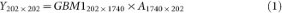Y is the PC score matrix for GBM1. A is the PC vector matrix.

2. 2

Next, we simulate a random score matrix YN where column i is populated with random values in a normal distribution with zero mean and standard deviation equal to that of column i in Y.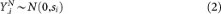where si is the standard deviation of Y.i and i = {1, …, 202}.

3. 3

Multiplying YN with the transpose of A yields QN, one of the pcNormal simulations.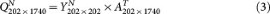4. 4

We repeat steps 2 and 3 50 times to obtain a collection of 50 pcNormal simulations.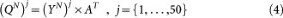### Choosing a representative null dataset from pcNormal

A representative dataset, called Sim25, is chosen from pcNormal as having clustering signals closest to the median of the 50 datasets, as measured by the average of positive silhouette widths and the fraction of negative silhouette widths. Let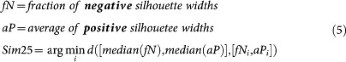where [fNi, aPi] is the silhouette width statistics for simulation i {1, …, 50} and d is the Euclidean distance function in the [fN, aP] space.

### Choosing nine pcNormal simulations for validation by most discriminant genes

The Euclidean distance of the (aP,fN) pair to the median of these quantities in the pcNormal cohort was computed for each of the 50 simulations and ranked from lowest to highest. Every 5th dataset was selected among the ranked simulations, such that the [6, 11, 16, 21, 26, 31, 36, 41, 46] ranked datasets were chosen. This ensures that nine datasets cover the entire range of clustering strength in pcNormal.

### Generating positive datasets for comparing the ability to find true K

To generate a positive dataset with K clusters, we first ran k-means clustering on Sim25 with the designated K in the range of 2–6. Next, we computed the centroids of the PC scores for each of the K clusters and added a known fraction of the centroid coordinates (i.e. the pull-apart degree, denoted as a positive scalar, "a") to the original PC scores of each sample in the corresponding cluster. Next, we multiplied the resulting PC scores from all clusters by the original principal component vectors of Sim25 so that the pull-apart datasets preserve the initial gene-gene correlation structure (with the caveat that increasing a values would gradually increase the gene-gene correlation).

Algorithmically, we execute the following steps for this procedure:

1. 1

Use principal component analysis to obtain the eigenvector matrix A as before.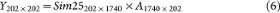2. 2

Use k-means to find K clusters in Sim25, assign each sample si (i = 1, …, 202) into one of K classes. The set of samples in class k(k = 1, …, K) is denoted as Ek

3. 3

For each set Ek, compute the centroid Ck of PC scores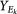4. 4

For each set Ek and for a given pull-apart degree a, compute pulled-apart score matrix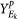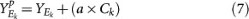5. 5

Multiply Yp with AT to obtain the pulled-apart dataset Xp.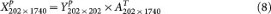### Base method for consensus clustering: K-means

Given a set of observations (x1, x2, …, xn) where each observation is a d-dimensional real vector, k-means clustering aims to partition the n observations into k sets (kn), S = {S1, S2, …, Sk} so as to minimize the within-cluster dispersion: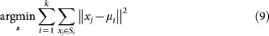where μi is the mean of points in Si36.

The method starts with k arbitrary cluster centers. Each search step consists of assigning each observation to its nearest cluster center and updating the centers of the clusters according to the observations assigned to each cluster. The procedure is repeated until the cluster assignment no longer changes.

### Five ways to measure clustering signals and determine K

#### Empirical CDF

For a given consensus matrix M, the corresponding empirical cumulative distribution (CDF) is defined over the range [0, 1] as follows: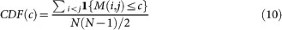where 1{…}denotes the indicator function, M(i, j) denotes entry (i, j) of the consensus matrix M, N is the number of rows (and columns) of M and c is the consensus index value9.

#### Proportional area change under CDF (Δ(K))

The changes of CDF as K increases provide evidence for finding the optimal number of clusters. A CDF curve that closely describes a three-phase step function is indicative of a higher cluster stability. A method for using this information is to select the largest K that induces a large enough increase in the area under the CDF9, which is defined as: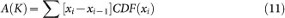The progression, in turn, can be visualized by plotting the proportion increase Δ(K) in the CDF area as K increases. Δ(K) is computed as follows: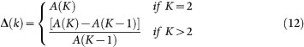The optimal K according to Δ(K) is the K where the ‘elbow’ occurs in the Δ(K) vs. K plot. This is a subjective criterion and in cases where the elbow occurs at a Δ(K) value very close to zero, the optimal K can also be considered to be the K before the elbow occurs or the K where Δ(K) reaches its maximum. In Figure 6, we adopted this last decision rule due to the elbow rule not being amenable to automatization.

### Silhouette width

The silhouette widths of a clustering result16 have been applied to report clustering strength and to find the optimal number of clusters K. For an object i in the dataset, let A denote the cluster to which it is assigned and define

a(i) = average dissimilarity of i to all other objects of A

For each of the clusters CA, calculate

d(i,C) = average dissimilarity of i to all objects of C

Then select the smallest of d.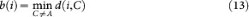The silhouette width of object i is defined as: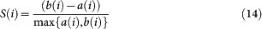It can be seen that S(i) lies between −1 and +1.

In Supplementary Figure 3b we compare GBM1 with the null simulations according to two summary statistics derived from silhouette widths. One is the "fraction of samples with negative silhouette widths". A negative silhouette width indicates that the sample is likely to have been assigned to the wrong cluster. The second statistic is the "average of positive silhouette widths". Higher values of this statistic indicate stronger cluster separation.

### GAP-statistic

The GAP-statistic provides an estimate for the number of clusters in a dataset by comparing the within-cluster dispersion Wk with that expected under an appropriate reference null distribution (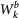where b {1,2, …, B})17. We first computed Wk for each K ≥ 2. We have not included K = 1 to ensure comparability across all methods tested here; methods such as CDF and silhouette width do not allow an inference of K = 1.

For the reference distribution, there are two alternative algorithms: GAP-unif and GAP-PC. For the former, the null datasets are generated from a uniform distribution over the range of each observed feature; and for the latter, they are generated from a uniform distribution over a box aligned with the principal components of the centered design matrix. The first approach has the advantage of simplicity, but the second approach can factor in the shape of the data distribution17. We chose the latter as it can take into account the empirical gene-gene correlation.

We generated B = 40 reference datasets using GAP-PC. Next, we computed the within-cluster sum of squares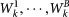for each reference dataset and estimated the gapk statistic with the formula: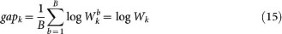The standard error for this quantity, sk, was then computed as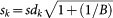where sdk is the uncorrected sample standard deviation of the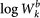quantities with b {1,2, …, B}.

The optimal K is the smallest K for which the GAP score is larger than the lower bound for K+1; where the lower bound is defined as the GAP score minus the standard error for that particular K value:

optimal K = smallest K such that gapkgapk+1sk+117

### Modified GAP-PC:

The original GAP-PC decision rule for the optimal K is to choose the smallest K where the gapk score is larger than the lower bound for K+1. Our modified, more intuitive decision rule is to declare the K value with the highest gapk score as the optimal K.

### CLEST

CLEST15 is a resampling-based method that randomly partitions the original dataset into a learning set and a test set. The former is used to build a K-cluster classifier, which is applied to partition the latter (the test set) in supervised assignment (such as DLDA37). The test set is also partitioned independently with the same unsupervised clustering algorithm as applied to the learning set. The concordance between the supervised and unsupervised partitions is summarized by measures such as the Fowlkes-Mallows (FM) index, for which a higher value indicates a stronger agreement of clustering results.

The observed concordance for each K is denoted as tk. Its estimated expected value under the null hypothesis of K = 1, namely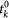, is then subtracted from tk to obtain the dk statistic. Among the K values that satisfy a pre-specified dmin criterion (here dmin = 0.05), the optimal K is the one with maximum dk. If none of the tested K values satisfy the pre-specified criteria, the optimal K is concluded to be 1.

### A new way to infer optimal K using CC: Proportion of Ambiguous Clustering (PAC)

The empirical CDF plot has consensus index values on the x-axis and CDF values on the y-axis. PAC is defined as the fraction of sample pairs with consensus index values falling in the intermediate sub-interval (x1, x2) [0, 1]. x1 and x2 are data-dependent thresholds, but will generally be chosen near 0 and 1 respectively. In our implementation, x1 = 0.1 and x2 = 0.9. Since CDF(c) corresponds to the fraction of sample pairs with consensus index values less than or equal to c as explained in the “Empirical CDF” section above, PAC is given by CDF(x2) - CDF(x1). A low value of PAC indicates a flat middle segment, allowing inference of the optimal K by the lowest PAC.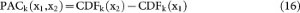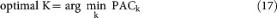### LCE Pseudo-code

LCE was implemented using functions from the LinkCluE package38.

We set N = 202 (Number of samples) and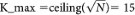.

Run 1: Fixed K. Execute step 1–5.

Step 1: Generate cluster ensemble from k-means runs with 10 different random seeds and fixed K where K = K_max.

Step 2: For ensemble from Step 1, create link-based similarity matrix using CTS (referred to as WCT in Iam-on et al.24) and decay factor 0.8.

Step 3: Partition the similarity matrix from Step 2 with a consensus function to assign samples into K_final clusters.

‐ Consensus function alternatives are average-, single- and complete-linkage HC

‐ Vary K_final in 2:6

Step 4: Evaluate quality of clusters using internal validity measures Davies-Bouldin (DB) and Dunn index. The DB and Dunn indices were calculated by comparing the partition from Step-3 and the k-means partition of the input data.

Step 5: Optimal K is the K_final with the best internal validity measure (highest Dunn index or lowest Davies-Bouldin index)

Run 2: Random K

‐ In Step 1, use random K in 2:K_max instead of fixed K. Then, execute step 2–5 as above.